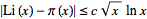# Prime Number Theorem: the meaning of the limit

• I
• DaTario

#### DaTario

Hi All.

I have a doubt concerning the limit:

$$\lim_{n \to \infty} \frac{\pi (n)}{Li(n)} = 1$$.

This mathematical statement does not imply that both functions converge to the same value. The main reason is that both tend to infinity as n tend to infinity. I would like to ask you if it is correct to infer that when n tends to infinity, ##\pi (n) ## and ## Li(n) ## grow at the same rate, possibly being separed by a constant.

If I am correct, is this constant known?

Best regards,

DaTario

How do you want to interpret the growth rate of ##\pi(n)##? It is a step function, which is discontinuous and hence constant except at a countable collection of points, at which it is not differentiable. But most interpretations of growth rate involve differentiation.

This mathematical statement does not imply that both functions converge to the same value. The main reason is that both tend to infinity as n tend to infinity. I would like to ask you if it is correct to infer that when n tends to infinity, ##\pi (n) ## and ## Li(n) ## grow at the same rate, possibly being separed by a constant.
The limit statement is even weaker. As an example: $$\lim_{n \to \infty} \frac{\ln (n) + \ln (\ln (n))}{\ln(n)} = 1$$
The difference between numerator and denominator diverges, but the ratio still goes to 1.

For this particular case, it is known that ##\pi(n) > Li(n)## and ##\pi(n) < Li(n)## switch infinitely often, the first time before 10371. Looks like there is no upper bound on the absolute deviation.

Thank you, mfb.

Answering andrewkirk, the function ##\pi (n)## is forced, by its definition, to increase by one unity at a time. As is goes to infinity, the relative weight of this discreteness goes to zero, allowing one to consider it as a continuous function. Its logarithmic nature also allows us to trust that no great departure from smoothness may happen for high values of ##n##. So the derivative of ##\pi(n)## may be taken as the average of:
$$\frac{\pi(n+1) - \pi(n)}{(n+1) - n}$$

for, say, a million of values of ##n## located at the vicinity of the point whose derivative we wish to calculate.
Note that our region of interest is ##n## large.

(someone, please correct me if I am wrong...)

Best wishes,

DaTario

There is no need to smoothen the function arbitrarily.

the function ##\pi (n)## is forced, by its definition, to increase by one unity at a time. As is goes to infinity, the relative weight of this discreteness goes to zero, allowing one to consider it as a continuous function. Its logarithmic nature also allows us to trust that no great departure from smoothness may happen for high values of ##n##. So the derivative of ##\pi(n)## may be taken as the average of:
$$\frac{\pi(n+1) - \pi(n)}{(n+1) - n}$$

for, say, a million of values of ##n## located at the vicinity of the point whose derivative we wish to calculate.
Let's formalise that. Your definition of growth rate is then

$$G(n)=10^{-6}\times \sum_{k=1}^{1000000}\frac{\pi(n-k+1)-\pi(n-k)}{(n-k+1)-(n-k)}= 10^{-6}\times\Big(\pi(n)-\pi(n-1000000)\Big)$$

That will not attain any limit as ##n\to\infty## because it will be 0 for many large ##n## but ##10^{-6}## for some and, rarely, ##m\times 10^{-6}## for values of ##m## greater than 1.

The growth rate of ##Li(n)## is ##H(n)\equiv\frac d{dn}\int_2^n\frac1{\log t}dt= \frac1{\log n}##, which smoothly tends towards a limit of zero as ##n\to\infty##. So neither ##G(n)-H(n)## nor ##\frac{G(n)}{H(n)}## has a limit as ##n\to\infty##.

Perhaps if we try to make a linear regression with a million of points of ##(n,\pi(n))##, with n ranging from ##n_0 - 5 \times 10^5 ## to ##n_0 + 5 \times 10^5 ##.
I would also accept a polynomial interpolation or a convolution with some gaussian.My point is that I was taking the limit of the theorem as an inference that both function would be identical as n tends to infinity. I now see that what seems to happen is very different from this.

I now see that what seems to happen is very different from this.
Right. There are always points where the functions differ by more than 10, or more than 100, or probably even more than a million. Removing the steps of 1 doesn't help to get convergence.It is an upper limit on the difference, but that limit goes to infinity. It is sufficient to see that the ratio converges to one, but it doesn't tell us if it has a fixed upper limit.

Yes.

Thank you.

Basically they try and use it to get the idea of how the prime counting function behaves.

The better the approximation - the better you can predict what the primes are.

The prime counting function though - is often elusive because of how "random" it seems.

Thank you, chiro.